• 手机端手机版芯视频 APP微信公众号维库官方抖音微信头条号
• |

# 评估传输线中的导体和介电损耗

### 传输线模型中的有损分量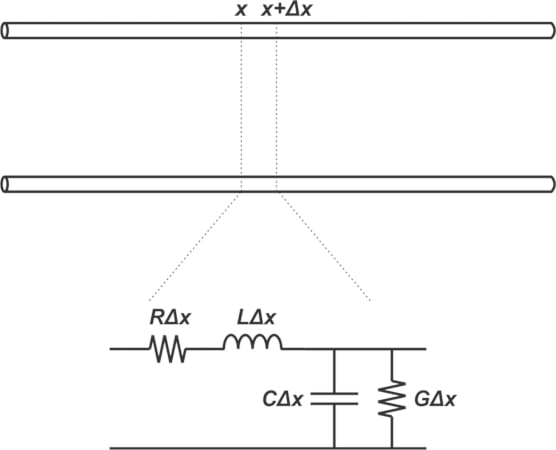##### 图 1.两导体传输线的等效电路。图片由 Steve Arar 提供

G=Ctan(δ)ω$G=Ctan(δ)ω$

### 有损线方程

$Z0=R+jLωG+jCω$

##### 等式2。

$γ=α+jβ=(R+jLω)(G+jCω)$

##### 等式 3。

• 衰减常数 ? 是 γ 的实部，以每米奈培为单位进行测量。
• 相位常数 β 是 γ 的虚部，以弧度/米为单位测量。它描述了每单位长度的相移。

$v(x,t)=Ae?αxcos(ωt?βx)$

### 简化 ? 以获得低损耗线路

$G=0.02×Cω$

##### 等式 5。

$右?LωAndG?Cω$

##### 等式 6。

$Z0≈LC$

##### 等式 7。

$γ=α+jβ=（右+jLω）（G+jCω）=jωLC（1+右jLω）（1+GjCω）$

##### 方程 8.

，使用 x ? 1 的近似 $$\sqrt{1~+~x}~\simeq~1~+~\frac{x}{2}$$ 可以证明：

$γ=α+jβ≈12（右Z0+GZ0）+jωLC$

### 分贝和奈珀斯

• 分贝。表达两个电压之比的常见方法是分贝以 10 为底，并使用 log 10 (V 1 /V 2 )计算。
• 内珀斯。更罕见的是，电压比以奈佩尔表示，它使用以e为底的自然对数。Nepers 使用 ln(V 1 /V 2 ) 计算。

##### 公式 11。

$αC=4.34×右Z0$

##### 公式 12。

$αd=4.34×GZ0$

### 介电损耗和导体尺寸

$Z0=εrC×C$

##### 公式 15。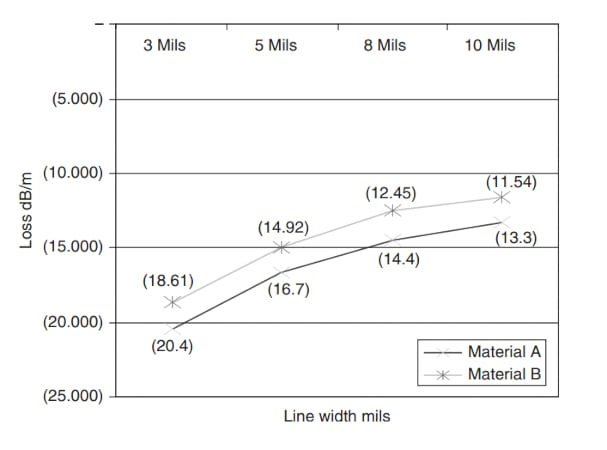##### 图 2. 两种材料的损耗与走线宽度的关系。图片由克莱德·库姆斯提供

$右AC≈ρpδΩ/米$

### 损耗随频率的变化

$δ=1πFμσ$

##### 公式 17。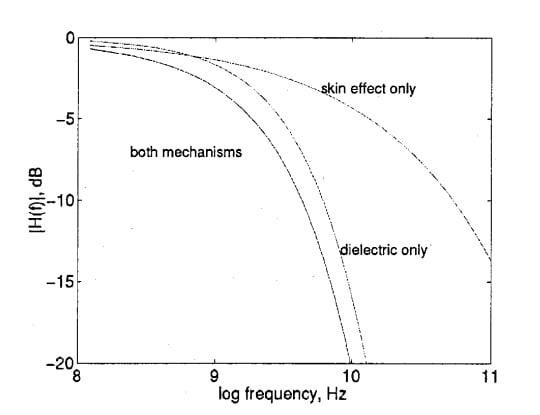##### 图 3.  40 厘米走线的导体损耗、介电损耗以及两者的综合影响。图片由C. Svensson提供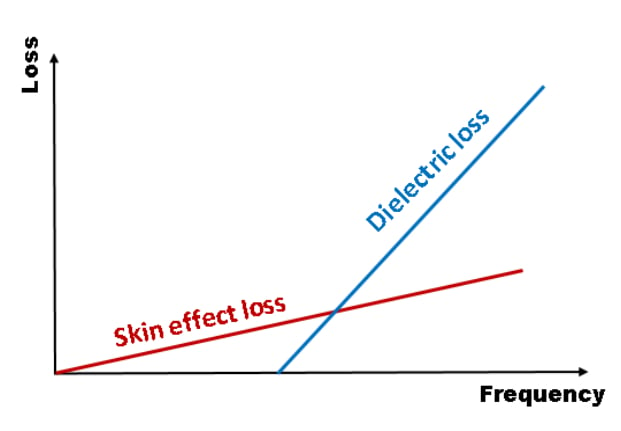##### 图 4. 集 肤效应损耗和介电损耗。图片由Y. Liao提供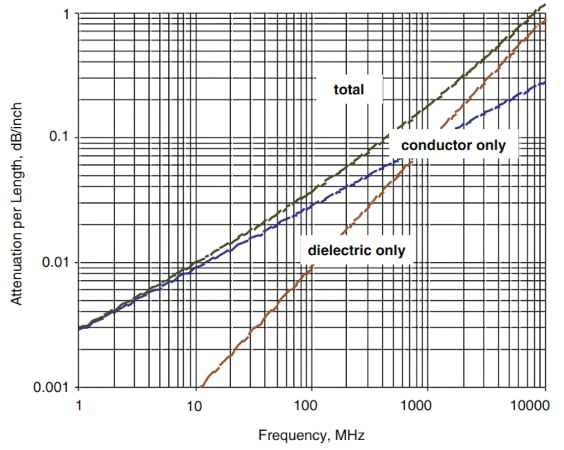### 传输线的其他性能区域

$ZC≈右jωC$

### 版权与免责声明0571-85317607

9:00-17:30>>